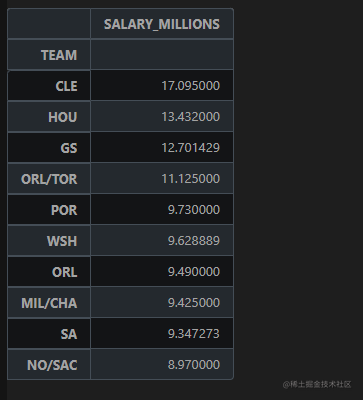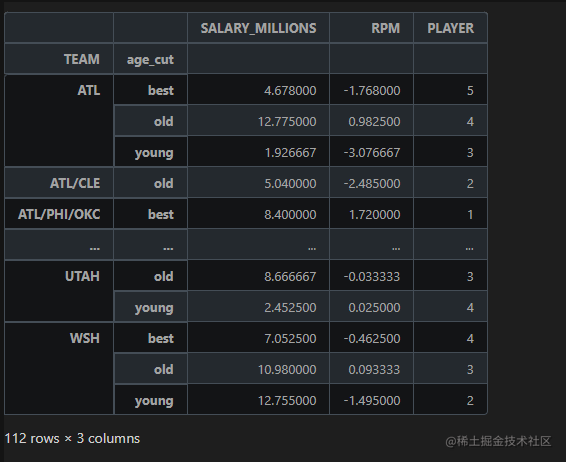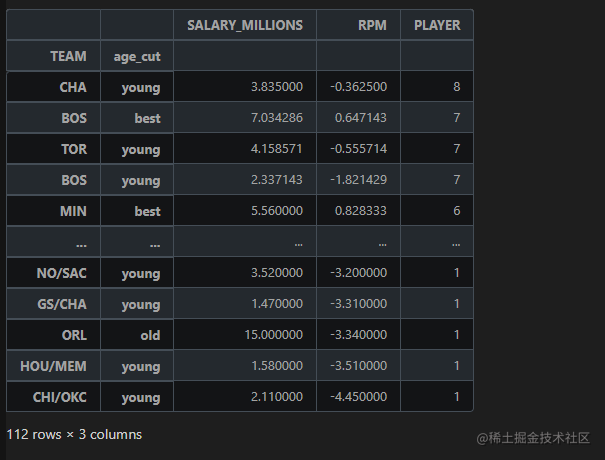# 1. 獲取資料集

### 1.1.2 球隊薪資排序

groupby：分組函式

agg是一個聚合函式，使用指定軸上的一個或多個操作進行聚合。在Pandas中可以利用agg()對SeriesDataFrame以及groupby() 後的結果進行聚合操作。 常用函式如min,max,median,std,count,size,sum等，直接用函式名加引號" "即可，如果有多個函式，用逗號隔開;

• 對NBA球隊進行分組，然後將每個組的SALARY_MILLIONS進行聚合，並且計算平均值。
• 對分組聚合後的資料進行降序排序，展示前十名資料
• ascending=False：降序排列
• sort_values：對指定列排序 ```python NBA_TEAM = NBA.groupby(by="TEAM").agg({"SALARY_MILLIONS": np.mean})

# 看前十名

```## 1.2 球隊年齡結構

### 1.2.1 分組聚合

• 分了兩個組：球隊和年齡段
• 聚合了三個指標：薪水，效率值，上榜球員數量
• 我們先分組聚合，再進行排序

```python NBA_rpm = NBA.groupby(by=["TEAM","age_cut"]).agg({"SALARY_MILLIONS": "mean", "RPM": "mean", "PLAYER":"size"}) NBA_rpm```### 1.2.2 排序

• 先以球員數量PLAYER排序
• 若PLAYER數量相同，再使用效率值RPM排序
• 都是降序排列

```python NBA_rpm.sort_values(by=["PLAYER", "RPM"], ascending=False)``` 執行結果如下圖所示：# 2. 球隊資料視覺化

## 2.1 繪製箱形圖

• 篩選出指定球隊的球員樣本
• 繪製箱形圖
• 指定箱型圖的x軸和y軸

### 2.1.1 獲取指定資料

• 我們想獲取指定球隊的資料
• 以表的形式返回 ```python

# 獲取需要的資料

NBA_TEAM2 = NBA[NBA.TEAM.isin(['GS','CLE','SA','LAC','OKC'])] ```

### 2.1.2 繪製箱形圖

• 三張圖放在一個畫布裡面
• 希望檢視三個特徵
• x軸為不同的TEAM
• y軸為對應的特徵 ```python sns.set_style("whitegrid") plt.figure(figsize=(20,10))

# 進行相應的繪圖

plt.subplot(3,1,1) sns.boxplot(x='TEAM', y='SALARY_MILLIONS', data = NBA_TEAM2)

plt.subplot(3,1,2) sns.boxplot(x='TEAM', y='AGE', data = NBA_TEAM2)

plt.subplot(3,1,3) sns.boxplot(x='TEAM', y='MPG', data = NBA_TEAM2)

plt.savefig("./箱形圖.png") ```